# Algebraic Data Types

Episode #4 • Feb 19, 2018 • Free Episode

What does the Swift type system have to do with algebra? A lot! We’ll begin to explore this correspondence and see how it can help us create type-safe data structures that can catch runtime errors at compile time.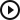Algebraic Data Types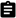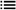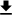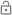This episode is free for everyone.

### Subscribe to Point-Free

Access all past and future episodes when you become a subscriber.

See plans and pricing

### Introduction

Today we wanna talk about a link between two worlds: Swift types and algebra. We all have familiarity with both of these worlds, but it turns out they are related in a very deep, beautiful way. Using this correspondence we can better understand what adds complexity to our data structures, and even use intuition we have from algebra to better sculpt our data so that impossible states are not representable, and hence do not compile!

### The algebra of structs

First, let’s look at something simple enough: structs. In fact, this particular struct:

``````struct Pair<A, B> {
let first: A
let second: B
}
``````

This is kinda the most generic, non-trivial struct one could make. It has two fields, and each just holds a piece of generic data. Let’s do something a little strange, and for particular values of `A` and `B`, let’s count how many values `Pair<A, B>` holds:

``````Pair<Bool, Bool>(first: true, second: true)
Pair<Bool, Bool>(first: true, second: false)
Pair<Bool, Bool>(first: false, second: true)
Pair<Bool, Bool>(first: false, second: false)
``````

`Pair<Bool, Bool>` holds exactly four values. It is impossible to construct any other value that would be a valid, compiling Swift program. Let’s try another. I’m gonna cook up a lil type that has exactly three values and count its pairs with `Bool`:

``````enum Three {
case one
case two
case three
}
``````

How many pairs can we build with `Bool` and `Three`?

``````Pair<Bool, Three>(first: true, second: .one)
Pair<Bool, Three>(first: true, second: .two)
Pair<Bool, Three>(first: true, second: .three)
Pair<Bool, Three>(first: false, second: .one)
Pair<Bool, Three>(first: false, second: .two)
Pair<Bool, Three>(first: false, second: .three)
``````

OK this has six values! Interesting!

There’s a strange type in Swift called `Void`. In fact it’s strange for at least two reasons. For one, you can refer to the type and the value in the same way:

``````_: Void = Void()
_: Void = ()
_: () = ()
``````

Well, that leads us to the other strange thing. `Void` only has one value! Because of that, the material substance of `()` doesn’t matter at all. It’s just a value that represents we have the thing in `Void`, but you can’t actually do anything with `()`. This is also why functions that have no return value secretly return `Void` even if not explicitly specified:

``````func foo(_ x: Int) /* -> Void */ {
// return ()
}
``````

The Swift compiler will just go in after you and `return ()`.

Let’s try plugging `Void` into our pair and see what happens:

``````Pair<Bool, Void>(first: true, second: ())
Pair<Bool, Void>(first: false, second: ())
``````

It only holds two values.

What about a pair of `Void` and `Void`?

``````Pair<Void, Void>(first: (), second: ())
``````

Only 1 value!

Interesting! We had no choice but to plug in `()` for the second field, and so it didn’t really change the type much.

There is yet another strange type in Swift: `Never`. Its definition is simple enough:

``````enum Never {}
``````

What does this mean? It’s an enum with no cases. This is the so-called “uninhabited type”: a type that contains no values. There is no way to do something like:

``````_: Never = ???
``````

There’s nothing we can put here and have Swift compile this program.

So what happens when we plug `Never` into `Pair`?

``````Pair<Bool, Never>(first: true, second: ???)
``````

There’s nothing we can put into `???`!

The `Never` type also gets special treatment by the compiler, in which a function that returns `Never` is known to be a non-returning function. For example, the `fatalError` function returns `Never`. The compiler knows that all lines and branches of code after the execution of this statement will never happen, and can use that to prove exhaustiveness.

With all of these examples in mind, what is the relationship between the number of values in `A` and `B` and the number of values in `Pair<A, B>`?

``````// Pair<Bool, Bool>  = 4
// Pair<Bool, Three> = 6
// Pair<Bool, Void>  = 2
// Pair<Void, Void>  = 1
// Pair<Bool, Never> = 0
``````

A pattern’s beginning to emerge: multiplication!

``````// Pair<Bool, Bool>  = 4 = 2 * 2
// Pair<Bool, Three> = 6 = 2 * 3
// Pair<Bool, Void>  = 2 = 2 * 1
// Pair<Void, Void>  = 1 = 1 * 1
// Pair<Bool, Never> = 0 = 2 * 0
``````

`Pair` causes the number of values to multiply, i.e. the number of values in `Pair<A, B>` is the number of values in `A` times the number of values in `B`.

There’s another algebraic interpretation of this phenomenon: logical conjunction, a.k.a. and. The `Pair` type is encapsulating what it means to take the “and” of two types, i.e. a value of `Pair<A, B>` is precisely a value of type `A` and a another value of type `B`.

And this is true of any struct and tuple, not just `Pair`. Let’s look at an example:

``````enum Theme {
case light
case dark
}

enum State {
case highlighted
case normal
case selected
}

struct Component {
let enabled: Bool
let state: State
let theme: Theme
}
``````

What’s the algebra of `Component`?

``````// Bool * Theme * State = 2 * 3 * 2 = 12
``````

`Component` has twelve values!

With this intuition, let’s wipe away all of the names of the types, and just focus on what data is stored in the fields. To do that, we are going to create a notation that is not valid Swift code, but will allow us to more compactly see the algebra we are uncovering here. So where we used to write `Pair<A, B>` we are now simply going to write `A * B`. Indeed this looks strange, but it is only to help guide our intuition:

``````// Pair<A, B>        = A * B
// Pair<Bool, Bool>  = Bool * Bool
// Pair<Bool, Three> = Bool * Three
// Pair<Bool, Void>  = Bool * Void
// Pair<Bool, Never> = Bool * Never
``````

We call `A * B` the product of the types `A` and `B`. And now that we are thinking a little bit more abstractly, we can even loosen our intuition around `Pair<A, B>` being the literal multiplication of the number of elements in `A` and `B`. While that is indeed true for types with finitely many values, that doesn’t really help us with things like:

``````// Pair<Bool, String> = Bool * String
``````

We no longer get to talk about the number of values, because `String` has an infinite number, but we’re still allowed to think of this as multiplication.

We could even consider the following:

``````// String * [Int]
// [String] * [[Int]]
``````

We’re multiplying infinite types together!

Let’s take things a step further and wipe away the names from `Void`, `Never` and `Bool` and only represent those types by the number of values that are contained within.

``````// Never = 0
// Void = 1
// Bool = 2
``````

So now we aren’t even thinking about specific types, just abstract algebraic entities.

### The algebra of enums

OK, now we’ve seen that structs in Swift correspond to multiplication of types. But there’s a corresponding “dual”: addition! How’s this look like in Swift’s type system?

Well, turns out Swift has support for such a construction, and that’s precisely an `enum`! Let’s consider the most generic, non-trivial enum one could make:

``````enum Either<A, B> {
case left(A)
case right(B)
}
``````

Let’s take some of our earlier values and see how to construct some simple values from this type:

``````Either<Bool, Bool>.left(true)
Either<Bool, Bool>.left(false)
Either<Bool, Bool>.right(true)
Either<Bool, Bool>.right(false)
``````

We get four values again. What about `Three`?

``````Either<Bool, Three>.left(true)
Either<Bool, Three>.left(false)
Either<Bool, Three>.right(.one)
Either<Bool, Three>.right(.two)
Either<Bool, Three>.right(.three)
``````

This time we get five values. Hm! How about `Void`?

``````Either<Bool, Void>.left(true)
Either<Bool, Void>.left(false)
Either<Bool, Void>.right(Void())
``````

Three values!

And `Never`?

``````Either<Bool, Never>.left(true)
Either<Bool, Never>.left(false)
Either<Bool, Never>.right(???)
``````

This last example is particularly interesting. We saw that by taking a pair with `Never`, i.e. `Pair<A, Never>`, we made the pair uninhabited. However, with `Either` it just means that one case is uninhabited, but the other is free to take values in `Bool`.

Now we can see some algebra peeking through. So what’s the relationship between the number of values in `A` and `B` and the number of values in `Either<A, B>`?

``````Either<Bool, Bool>  = 4 = 2 + 2
Either<Bool, Three> = 5 = 2 + 3
Either<Bool, Void>  = 3 = 2 + 1
Either<Bool, Never> = 2 = 2 + 0
``````

From these examples we can see that the number of values in `Either<A, B>` is precisely the number of values in `A` plus the number of values of `B`. So `Either` directly corresponds to taking the sum of types. This is why enums are called “sum types.” We can also interpret `Either` from the perspective of logic like we did for `Pair`: the `Either` type encapsulates what it means to take the “or” of two types, i.e. a value of `Either<A, B>` is precisely a value of type `A` or a value of type `B`.

So, like before let us abstract away the idea of taking the sum of types by using a new notation that isn’t valid Swift but nonetheless will be helpful for developing our intuition.

``````Either<Bool, Bool>  = Bool + Bool  = 2 + 2 = 4
Either<Bool, Three> = Bool + Three = 2 + 3 = 5
Either<Bool, Void>  = Bool + Void  = 2 + 1 = 3
Either<Bool, Never> = Bool + Never = 2 + 0 = 2
``````

### Word of warning: Void

It’s worth noting that some languages (such as Haskell, PureScript, Idris) use `Void` to denote the uninhabited type (i.e., what Swift calls `Never`), and so could lead to some confusion if you look into those languages. And in fact, in some sense that’s a great name since “void” kinda seems like a space that has nothing in it!

Perhaps a better name for the type with one unique value would be something like `Unit`. We would define it as such:

``````struct Unit {}
let unit = Unit()
``````

This is nice because we now have a distinct name for the type `Unit` and the unique value `unit`. Another nice thing about having an actual struct type for `Unit` is that we get to extend it:

``````extension Unit: Equatable {
static func == (lhs: Unit, rhs: Unit) -> Bool {
return true
}
}
``````

And now we are allowed to pass `unit` into functions that only want equatable value, which is cool. But that isn’t possible with `Void` in Swift. If you try to extend it you get this error:

``````Non-nominal type 'Void' cannot be extended
``````

The reason is that `Void` is defined as an empty tuple:

``````typealias Void = ()
``````

Tuples in Swift are non-nominal types, i.e. you don’t get to refer to them by name, only by structure. This is a very unfortunate thing in Swift that can hopefully some day be remedied.

### Empty structs vs. empty enums

But now we want to call out something very strange. Let’s look at the definitions of `Unit` and `Never` side-by-side:

``````struct Unit {}
enum Never {}
``````

Clearly there’s some symmetry here: an enum with no cases and a struct with no fields. By why does the enum with no cases have no values in it, yet the struct with no fields does have a value? It’s perfectly reasonable to maybe expect that `Unit` also has no values.

However can we get intuition to understand why this is the case?

Using our correspondence between Swift types and algebra, we can ask a related question that is perhaps easier to answer. We can ask ourselves, “What values are in the empty enum and empty struct?” and it’s equivalent to asking, “What is the sum and product of integers in the empty array?”

So, say we had an array of integers. How can we define the following functions:

``````func sum(_ xs: [Int]) -> Int {
fatalError()
}

func product(_ xs: [Int]) -> Int {
fatalError()
}

let xs = [1, 2, 3]
sum(xs)
product(xs)
``````

Well we definitely want to loop over the arrays and sum and multiply all the values together:

``````func sum(_ xs: [Int]) -> Int {
var result: Int
for x in xs {
result += x
}
return result
}

func product(_ xs: [Int]) -> Int {
var result: Int
for x in xs {
result *= x
}
return result
}
``````

This doesn’t currently compile because we haven’t given an initial value to `result`. But what should we choose? Well, to answer that question we need to understand what properties `sum` and `product` should satisfy, and that will force our hand as to what `result` needs to start at. The simplest property we would want to satisfy has to do with how `sum` and `product` behave with respect to concatenation of arrays:

``````sum([1, 2]) + sum() == sum([1, 2] + )
product([1, 2]) * product() == product([1, 2] + )
``````

Now, what if we used empty arrays?

``````sum([1, 2]) + sum([]) == sum([1, 2] + [])
product([1, 2]) * product([]) == product([1, 2] + [])
``````

This forces `sum([])` to be `0` and `product([])` to be `1`. There are no other choices. Therefore the empty sum is `0` and the empty product is `1`.

``````sum([1, 2]) + 0 == sum([1, 2] + [])
product([1, 2]) * 1 == product([1, 2] + [])

sum([]) == 0
product([]) == 1
``````

Now, transporting this concept back to the type world, we are naturally led to the statement that the “empty sum type” has no values (i.e. uninhabited) and that the “empty product type” has exactly one value! So we’ve used algebra to disentangle a really gnarly existential quandary!

### Algebraic properties

Now that we’ve built up some of the concepts to understand the correspondence between Swift types and algebra, let’s try to flex these muscles and see if we can get intuition on some type constructions at this higher level.

Let’s start easy. Recall that `Void` corresponds to `1`, and in the algebra world we know that multiplying by `1` doesn’t do anything. What does this look like in types?

``````// Void = 1
// A * Void = A = Void * A
``````

This means that using a `Void` value in the field of a struct has the net effect of essentially leaving the type unchanged.

On the other hand, `Never` corresponds to `0`, and we know that multiplying with it results in `0`. In the type world this look like:

``````// Never = 0
// A * Never = Never = Never * A
``````

So putting `Never` in a field of the struct has the net result of turning that struct into a `Never` type itself. It completely annihilates it.

But, adding `0` has a net result of leaving the value unchanged, and in types this corresponds to:

``````// A + Never = A = Never + A
``````

Let’s go the other way. Consider this type expression:

``````// 1 + A = Void + A
``````

In terms of `Either` this is:

``````// Either<Void, A> {
//   case left(())
//   case right(A)
// }
``````

So this is the type that has all of the values of `A` on the right side, and then this one special value `left(Void())` is adjoined. What native Swift type has this same shape? Optionals!

``````enum Optional<A> {
case none
case some(A)
}
``````

The `none` case corresponds to `left` (a case with no associated value is essentially the same as a case with a `Void` value), and the `some` case corresponds to `right`. So now we have seen:

``````// 1 + A = Void + A = A?
``````

Now, say you came across this expression:

``````// Either<Pair<A, B>, Pair<A, C>>
``````

Let’s see what it looks like in our notation:

``````// A * B + A * C
``````

Using basic algebra we understand how to factorize this into a simpler expression:

``````A * (B + C)
``````

And now this corresponds to a pair with an enum:

``````Pair<A, Either<B, C>>
``````

So here we see that algebraic intuition has led us to a simpler data structure.

On the other hand, if we simply flip the roles of `Pair` and `Either`, we have:

``````Pair<Either<A, B>, Either<A, C>>
``````

And in the math world:

``````// (A + B) * (A + C)
``````

This equation does not factorize anymore and so we cannot make it any simpler.

We could, of course, expand it out so that it equals:

``````// A * A + A * C + B * A + B * C
``````

And this is kind of like an enum with four cases, each case being a pair. That may not be what you want, but maybe you do, and you have the algebra to show you how to do it!

Every data structure that we talk about, if we just think of the data and none of the behavior we associate with the data, it’s all just sums of products: you start with a base enum of cases, and each case you have a bunch of products, which may in turn contain more sums and products.

### What’s the point?

We’ve written a bunch of pseudocode that isn’t even valid Swift, all it can do is guide our intuition. Have we gotten any benefit out of this?

Let’s look at a method on `URLSession`.

``````// URLSession.shared
//   .dataTask(with: url, completionHandler: (data: Data?, response: URLResponse?, error: Error?) -> Void)
``````

The completion handler gives us back three values that are all optional. This is a product type with 3 fields. Swift Tuples are just products. Let’s express it algebraically:

``````// (Data + 1) * (URLResponse + 1) * (Error + 1)
``````

This looks a little strange. What happens if we fully expand it?

``````// (Data + 1) * (URLResponse + 1) * (Error + 1)
//   = Data * URLResponse * Error
//     + Data * URLResponse
//     + URLResponse * Error
//     + Data * Error
//     + Data
//     + URLResponse
//     + Error
//     + 1
``````

There are a lot of representable states here that don’t make sense. They even jump out on each line. We can get `URLResponse * Error`, while `URLResponse` should never be inhabited at the same time as `Error`. We can also get `Data * Error`, which also makes no sense. We can also get `1`, which is just `Void`, or in this case where everything value is `nil`. And we can also get everything: `Data * URLResponse * Error`, which should never happen.

### Correction

It was brought to our attention by one of our viewers, Ole Begemann, that it is in fact possible for `URLResponse` and `Error` to be non-`nil` at the same time. He wrote a great blog post about this, and we discuss this correction at the beginning of our follow up episode, Algebraic Data Types: Exponents.

When you work with this interface, you may notice that when you `if let` over the cases you expect, you inevitably end up with a branch that you need to `fatalError`, and just hope it never gets called.

Let’s use our new intuitions to represent just what we want:

``````// Data * URLResponse + Error
``````

What’s this look like with our types?

``````Either<Pair<Data, URLResponse>, Error>
``````

In fact, the Swift community has embraced a type that allows us to handle these kinds of states, the `Result` type.

``````// Result<(Data, URLResponse), Error>
``````

And in this case, rather than use our `Pair`, we can use a simple tuple to represent the product of `Data` and `URLResponse`.

By using the proper type in the completion callback, we have greatly reduced the number of invalid states that are allowed at compile time, thus simplifying the logic needed in the callback.

Let’s consider the `Result` type further. What if we’re using an API that returns `Result` but with a particular operation that can never fail? We can specify that our error type is `Never`!

``````// Result<A, Never>
``````

Now we can be sure that the error case is uninhabitable.

And what if we’re dealing with an asynchronous API that supported cancellation? How could we add that cancellation case to our `Result`?

``````// Result<A, Error>?
``````

We just make it optional!

Seeing how we can both wrangle complexity and lead ourselves naturally to types that better fit our needs makes it clearer how algebraic intuitions can improve our everyday code. We also see that while structs have lightweight versions in tuples, maybe `Either` is a lightweight enum that belongs in our daily arsenal. Let’s not be afraid of `Either`! To be afraid of `Either` but not be afraid of tuples, it’s like saying that you’re afraid of addition, but not multiplication. Or it’s like saying you’re afraid of “or”, but not “and.” We don’t program in a way in which we only use multiplication (`*`) and “and” (`&&`). We allow ourselves to use addition (`+`) and “or” (`||`). So let’s get comfortable with sum types and `Either`!

We’ve only just begun on this algebraic journey. We still haven’t seen how the type system can represent other concepts, like exponentiation! What does one type to the power of another look like? But that’ll have to wait till next time. Stay tuned!This episode is free for everyone.

### Subscribe to Point-Free

Access all past and future episodes when you become a subscriber.

See plans and pricing

### Exercises

1. What algebraic operation does the function type `(A) -> B` correspond to? Try explicitly enumerating all the values of some small cases like `(Bool) -> Bool`, `(Unit) -> Bool`, `(Bool) -> Three` and `(Three) -> Bool` to get some intuition.

Solution

For every input, a function must assign a single output. Viewed this way, we can enumerate implementations for the cited functions:

`(Bool) -> Bool`

• `true -> true, false -> true`
• `true -> false, false -> false`
• `true -> false, false -> true`
• `true -> true, false -> false`

⇒ four inhabitants of `(Bool) -> Bool`.

`(Unit) -> Bool`

• `Unit() -> true`
• `Unit() -> false`

⇒ two inhabitants of `(Unit) -> Bool`.

`(Bool) -> Three`

• `true` and `false` both need to be mapped onto `Three` ⇒ we have three choices for `true` and three for `false` ⇒ nine inhabitants of `(Bool) -> Three`.

`(Three) -> Bool`

Each member of `Three``.one`, `.two`, and `.three`—need to be mapped onto `Bool` ⇒ we have two choices per case, totaling at `2*2*2 = 2^3 = 8` inhabitants of `(Three) -> Bool`.

More generally, functions from `A -> B` correspond to the cardinality of `B` raised to `A`’s cardinality, i.e. `B^A`.

2. Consider the following recursively defined data structure:

``````indirect enum List<A> {
case empty
case cons(A, List<A>)
}
``````

Translate this type into an algebraic equation relating `List<A>` to `A`.

Solution

Since `List` is a sum type and the `List.cons` has two associated values (a tuple), we can expand its cardinality as follows:

`List<A> = 1 + A * List<A>`

And recursing,

``````List<A>
= 1 + A * (1 + A * List<A>)
= 1 + A + A*A * List<A>
= 1 + A + A*A * (1 + A * List<A>)
= 1 + A + A*A + A*A*A * List<A>
= 1 + A + A*A + A*A*A + A*A*A*A * ...
``````
3. Is `Optional<Either<A, B>>` equivalent to `Either<Optional<A>, Optional<B>>`? If not, what additional values does one type have that the other doesn’t?

Solution

`Optional<Either<A, B>> = (A + B) + 1 = A + B + 1`

`Either<Optional<A>, Optional<B>> = (A + 1) + (B + 1) = A + B + 1 + 1`

They’re not equivalent. `Either<Optional<A>, Optional<B>>` has one more inhabitant than `Optional<Either<A, B>>`.

We can think of `Optional<Either<A, B>>` as

``````case some(Either<A, B>), case none
``````

and `Either<Optional<A>, Optional<B>>`—when expanded—as

``````case some(Either<A, B>), case none, case other
``````

where the `other` case is the extra `1` in the equations above.

4. Is `Either<Optional<A>, B>` equivalent to `Optional<Either<A, B>>`?

Solution

`Either<Optional<A>, B> = (A + 1) + B = A + B + 1`

`Optional<Either<A, B>> = (A + B) + 1 = A + B + 1`

They’re equivalent!

5. Swift allows you to pass types, like `A.self`, to functions that take arguments of `A.Type`. Overload the `*` and `+` infix operators with functions that take any type and build up an algebraic representation using `Pair` and `Either`. Explore how the precedence rules of both operators manifest themselves in the resulting types.

Solution
``````enum Either<A, B> {
case left(A)
case right(B)
}

struct Pair<A, B> {
let first: A
let second: B
}

func * <A, B> (lhs: A.Type, rhs: B.Type) -> Pair<A, B>.Type {
return Pair<A, B>.self
}

func + <A, B> (lhs: A.Type, rhs: B.Type) -> Either<A, B>.Type {
return Either<A, B>.self
}
``````

To explore the precedence rules of both operators, let’s check the the type of the following expression:

``````Void.self + String.self * Int.self
``````

It’s `Either<Void, Pair<String, Int>>`, which is expected, since multiplication takes precedence over addition ⇒ `String` and `Int` would be `Pair`’d before joining under an `Either` with `Void`.

#### Making illegal states unrepresentable

Ole Begemann • Thursday Apr 26, 2018

Ole discusses the concept of “illegal states” in data types, and how to leverage the type-system to make those states completely impossible to construct. His article was inspired by a mistake we made in our episode on algebraic data types, which shows just how subtle this problem can be!